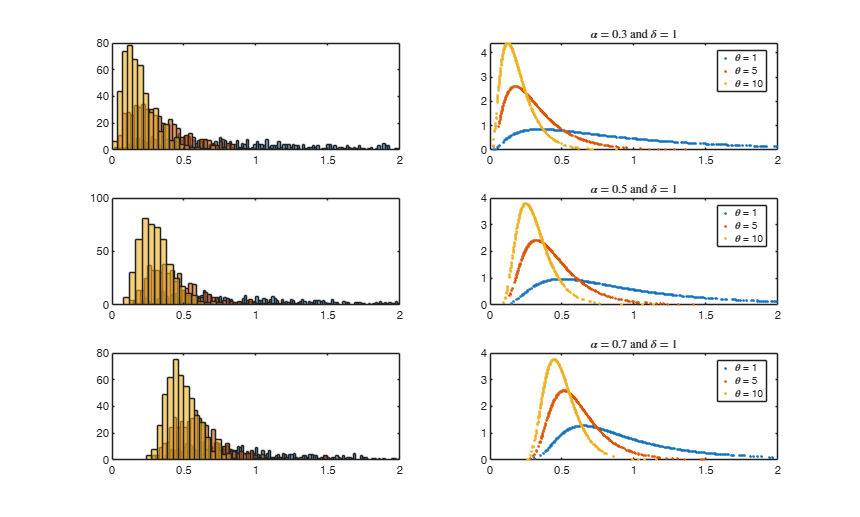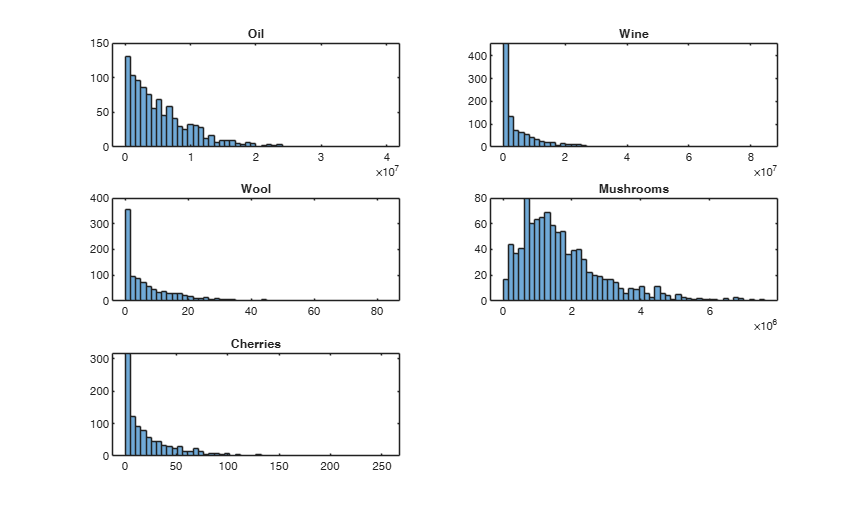# twdrnd

TWDRND generates random variates from the Tweedie distribution.

## Syntax

• Y=twdrnd(alpha,theta,delta)example
• Y=twdrnd(alpha,theta,delta,n)example

## Description

This function generates $n$ random numbers from a Tweedie distribution, with parameter space given by $﻿ (\alpha,\theta,\gamma) = \Big\{\; ]-\inf , 0[ \;\times\; ]0,\inf[ \;\times\; ]0,\inf[ \;\Big\} \cup \Big\{\; ]0 , 1[ \;\times\; [0,\inf[ \;\times\; ]0,\inf[ \;\Big\}$

The parameter space follows the Laplace transform formulation of Hougaard (1986) and Hougaard et al. (1997), The parameter $\delta$ crucially determines the characteristics of the Tweedie distribution. The family includes the continuous normal, gamma and inverse gaussian distributions.

$\delta = 0$ is for the gaussian.

$\delta = 1$ is for the poisson.

$\delta = 2$ is for the gamma.

$\delta = 3$ is for the inverse gaussian.

 Y =twdrnd(alpha, theta, delta) Plots of pdf and histogram of generated data for some parameter choices.

 Y =twdrnd(alpha, theta, delta, n) Examples from Barabesi et al (2016).

## Examples

expand all

###Plots of pdf and histogram of generated data for some parameter choices.

Parametrizations: alpha, theta, delta Hougaard (1986) and Hougaard et al. (1997).

% alpha, beta, gamma (Devroye (2009)).
% $\beta = \theta \delta^{1/alpha}$.
% $\gamma = \delta^{1/alpha}$.
alpha = [0.3 , 0.5 , 0.7];
theta = [1 , 5 , 10] ;
delta = [1 , 1 , 1];
beta  = theta.*delta.^(1./alpha);
gamma = delta.^(1./alpha);
n= 500;
hf = figure; af = gca(hf);
for i=1:3
hs1 = subplot(3,2,2*i-1);
hs2 = subplot(3,2,2*i);
leg = [];
for j=1:3
% generate random data vector X
X    = twdrnd(alpha(i),theta(j),delta(i),n);
bins = round(n/(2*theta(j)));
histogram(hs1,X,bins); hold(hs1,'on');
% use X to make the pdf
pdf = twdpdf(X,alpha(i),theta(j),delta(i));
plot(hs2,X,pdf,'.'); hold(hs2,'on');
xlim([hs1,hs2],[0,2]);
% to be used in the legend
assignin('caller',['leg' num2str(j)],['\theta = ' num2str(theta(j))]);
end
title(['$\alpha = ' num2str(alpha(i)) ' \mbox{ and } \delta = ' num2str(delta(i)) '$'] , 'interpreter','latex');
legend(leg1,leg2,leg3);
end###Examples from Barabesi et al (2016).

n = 1000;
pOil       = [-0.2697 , 0.291*10^(-6)  , 0.0282];
pWine      = [-0.0178 , 0.7802*10^(-7) , 0.3158];
pWool      = [-0.7380 , 0.145          , 0.2615];
pMushrooms = [-0.0936 , 1.27 *10^(-6)  , 0.6145];
pCherries  = [-0.3221 , 0.0324         , 0.2510];
param1 = pOil;          tit1 = 'Oil';
param2 = pWine;         tit2 = 'Wine';
param3 = pWool;         tit3 = 'Wool';
param4 = pMushrooms;    tit4 = 'Mushrooms';
param5 = pCherries;     tit5 = 'Cherries';
figure;
bins = round(n/20);
h1 = subplot(3,2,1);
al = param1(1) ; th = param1(2) ; de = param1(3) ;
t = twdrnd(al,th,de,n);
histogram(h1,t,bins);
title(h1,tit1);
h2 = subplot(3,2,2);
al = param2(1) ; th = param2(2) ; de = param2(3) ;
t = twdrnd(al,th,de,n);
histogram(h2,t,bins);
title(h2,tit2);
h3 = subplot(3,2,3);
al = param3(1) ; th = param3(2) ; de = param3(3) ;
t = twdrnd(al,th,de,n);
histogram(h3,t,bins);
title(h3,tit3);
h4 = subplot(3,2,4);
al = param4(1) ; th = param4(2) ; de = param4(3) ;
t = twdrnd(al,th,de,n);
histogram(h4,t,bins);
title(h4,tit4);
h5 = subplot(3,2,5);
al = param5(1) ; th = param5(2) ; de = param5(3) ;
t = twdrnd(al,th,de,n);
histogram(h5,t,bins);
title(h5,tit5);## Input Arguments

### alpha — Distribution parameter value. Non-zero value smaller than 1.

The location parameter of the Tweedie distribution. Default is alpha = 1.

Data Types: single| double

### theta — Distribution parameter value. Positive value.

The dispersion parameter of the Tweedie distribution. Default is theta = 0 (Dirac).

Data Types: single| double

### delta — Distribution parameter value. Positive value.

The power parameter of the Tweedie distribution. delta is such that the variance is $var(Y) = \theta * \alpha^\delta$. delta is greater than or equal to one, or less than or equal to zero. Default is delta = 1.

Interesting special cases are: the normal (delta=0), Poisson (delta=1 with theta=1), gamma (delta=2) and inverse Gaussian (delta=3). Other values of delta lead to cases that cannot be written in closed form, and the computation becomes difficult.

When 1 < delta < 2, the distribution is continuous for Y>0 and has a positive mass at Y=0. For delta > 2, the distribution is continuous for Y>0.

Data Types: single| double

### n — Number of random numbers to extract. Positive integer.

The sample size. Default is n = 1.

Example: n=100. 

Data Types: Scalar.

## Output Arguments

### Y —Random values extracted from the Tweedie distribution. Scalar or array

The random value(s). Scalar value | array of scalar values.

The Tweedie distribution is a very flexible family defined on positive numbers which is potentially useful for modeling data arising from a plethora of different frameworks. The family encompasses very heavy-tailed distributions, as well as light-tailed (i.e. exponentially-tailed) distributions. In addition, the Tweedie distribution may even model data with structural zeroes, since for some parameter values it reduces to a mixed distribution of Dirac mass at zero and an absolutely-continuous positive distribution. This feature is especially appealing when dealing with data arising from socio-economic phenomena, which indeed may produce structural zeroes - for example, owing to truncation of small values of the observations.

The Tweedie distribution is in the exponential family, and it is therefore useful in the generalized linear model framework. With this parameterization, the mean and variance for Tweedie variables are $\mbox{E}(X)=\mu$ and $\mbox{Var}(X)=\theta \mu ^ \delta$, respectively, where $\theta$ is the dispersion parameter and $\delta$ is an extra parameter that controls the variance of the distribution.

Except for the special cases mentioned above, the pdf for the Tweedie distribution does not have a closed form and can be expressed in terms of series; its evaluation then requires numerical approximations, which computationally are typically rather expensive . Dunn and Smyth (2005) use a finite series with a formula to determine the lower and upper indices to achieve the desired accuracy. Alternatively, Dunn and Smyth (2008) apply the Fourier transformation on the characteristic function.

Tweedie, M. C. K. (1984), An index which distinguishes between some important exponential families, "in Statistics: Applications and New Directions, Proceedings of the Indian Statistical Institute Golden Jubilee International Conference (J.K. Ghosh and J. Roy, eds.), Indian Statistical Institute, Calcutta", pp. 579-604.

Jorgensen, B. (1987), Exponential dispersion models, "Journal of the Royal Statistical Society, Series B", Vol.49, pp. 127-162.

Hougaard, P. (1986), Survival models for heterogeneous populations derived from stable distributions, "Biometrika", Vol. 73, pp. 387-396.

Hougaard, P., Lee M.T. and Whitmore, G.A. (1997), Analysis of overdispersed count data by mixtures of Poisson variables and Poisson processes, "Biometrics", Vol. 53, pp. 1225-1238.

Dunn, P. K. and Smyth, G. K. (2005), Series Evaluation of Tweedie Exponential Dispersion Model Densities, "Statistics and Computing", Vol. 15, pp. 267–280.

Dunn, P. K. and Smyth, G. K. (2008), Series Evaluation of Tweedie Exponential Dispersion Model Densities by Fourier Inversion, "Statistics and Computing", Vol. 18, pp. 73–86.

Barabesi, L., Cerasa, A., Perrotta, D. and Cerioli, A. (2016), Modeling international trade data with the Tweedie distribution for anti-fraud and policy support, "European Journal of Operational Research", Vol. 248, pp. 1031-1043.